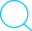19 Jan 2015

# Visual Odometry Estimation

### Visual odometry estimation

Step 1: Finding feature points and point correspondences

• 1.1 Use a feature detection algorithm (SIFT/SURF/BRISK/FREAK/ORB/FAST/HARRIS…) to detect the key points in each frame (In Matlab: `detectSURFFeatures` function)

• 1.2 Compute the feature descriptors for each key point ( In Matlab: `extractFeatures` function).

• 1.3 Find the indices of the matching keypoints between successive frames using a feature matching algorithm (In Matlab:`matchFeatures`function). to find the correspondences between two frames by comparing how close the descriptors of each of the keypoints in the two frames are.

Step 2: Reconstructing the 3D points

• 2.1 Since all the point correspondences obtained in the previous section need not necessarily be the inliers, use RANSAC to discard the outliers.

• 2.2 Estimate the Fundamental matrix. (In Matlab: `fRANSAC = estimateFundamentalMatrix(matchedPoints1,matchedPoints2,'Method', 'RANSAC', 'NumTrials', 2000, 'DistanceThreshold', 1e4);`Which uses matlab’s inbuilt RANSAC function.) It can be formulated as a least square problem can be solved using SVD!. (In Matlab:

``````for i=1:size(pts1,1)
A = [pts1(i,2)*P1(3,:)P1(2,:);
P1(1,:)pts1(i,1)*P1(3,:);
pts2(i,2)*P2(3,:)P2(2,:);
P2(1,:)pts2(i,1)*P2(3,:)];
[U S V] = svd(A);
h = V(:,end);
rp = P1*h;
worldPoints = [worldPoints h/h(4)];
``````

Where P1 and P2 are the camera projection matrices of camera 1 and camera 2 respectively. P1 was set to be K*[I |0]; P2 Was constructed using the baseline of the stereo pair and an Identity rotation.

• 2.3 Using the camera intrinsic parameters, the K matrix can be obtained and then the Essential matrix, E can be obtained from the fundamental matrix. (In Matlab: E = K’fRANSACK;)
• 2.4 The essential matrix E can be decomposed to obtain the 4 possible {R,t} pairs for the second frame in the pair of frames.
``````[U S V] = svd(E);
W = [0 1
0;1 0 0;0 0 1];
Z = [0 1 0;1
0 0;0 0 0];
R1 = U*W*V';
R2 = U*W'*V';
Tx = V*Z*V';
T1 = [Tx(3,2), Tx(1,3) , Tx(2,1)];
%T1 = U(:, 3);
T2 = T1;
P1 = R1; P1(:, 4) = T1;
P2 = R1; P2(:, 4) = T2;
P3 = R2; P3(:, 4) = T1;
P4 = R2; P4(:, 4) = T2;
``````
• 2.5 From the 4 possible projection matrices, the most reasonable projection matrix can be estimated. Use the one point correspondence to test each of the 4 possible projection matrices by reprojecting the pair of points in 3D and determining the depth of the point in both the left and the right camera. The P which gives a positive depth for the 3D point in both the camera’s view is taken to be the correct projection matrix.

• 2.6 After obtaining the correct P, re-projection error can be minimized using a RANSAC routine or using nonlinear least squares algorithm.

• 2.7 Once the camera projection matrices are estimated, we computed the 3D points using triangulation. Form the A matrix for the least squares problem using the 2D point correspondences and the projection matrices and use SVD to find the points in 3D space.

Step 3: Computing the successive transforms/projection matrices

• 3.1 From the 3D points obtained from the first image pair, the correspondences in the next successive image frame can be found and the transform/camera projection matrix can be computed using DLT . For the DLT, same approach of formulating the least squares problem using the 3D and 2D points and using SVD will help to find the solution.

• 3.2 The above process is iterated over all the successive images to obtain the incremental Rotation and translation matrices.